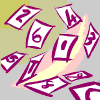What can you say about numbers that have exactly 2 factors?

What can you say about numbers that have exactly 3 factors?

Give some examples of numbers with 4 factors. What do they have in common?

Give some examples of numbers with 5 factors. What do they have in common?

What about numbers with 6 factors?

How many factors does 72 have?

Can you find a connection between the number of factors of 72 and the exponents in the expression giving the factorisation of 72 into primes?

$$72=2^3\times3^2$$

Give examples of some numbers with 12 factors. What is the smallest number with 12 factors.?

What is the smallest number with exactly fourteen divisors?

Tagged with:

### 3 Responses to Grades 9 to 11 – How Many Factors?

1.Sdumo Msindo says:

I gave this activity to my learners, some of them looked at all the factors of 11 -200. Other groups focussed on checking the pattern of finding the number of factors from the factors they already have.e.g 6 have 4 factors(1,2,3,6),then 6×2 is 12 meaning that you add 2 factors to those of 6 i.e(1,2,3,4,6,12),then for 24 add two factors to those of 12 i.e (1,2,3,4,6,8,12,24)this shows that 48 has 10 factors and 60 has 12 factors.

2.Cynthia Fries says:

Sounds like a busy lesson with lots of scope for differentiated learning. Your learners found one pattern chain, but it you continue doubling it goes 24, 48, 96 (not 60) and 96 does indeed have 12 factors. Did they look at 18 and 36? Doubling the previous number does not result in an extra 2 factors this time, but there is still a pattern to be found. Has anyone else found other chains? Or found a way of predicting the number of factors for any number?

3.Sdumo Msindo says:

Thanks Cynthia for that, but my learners only focussed on the factors of 12 and on multiplying by 12 although they started on 6m”. Their chain is : 12, 24, 36, 48, 60, 72,84, 96 and the smallest number with 12 factors is 60. They will be looking at the chain for 18, 36 etc.

Set your Twitter account name in your settings to use the TwitterBar Section.